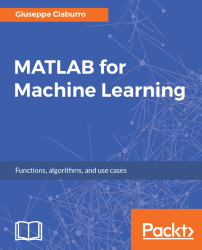•#### MATLAB for Machine Learning#### Overview of this book

MATLAB is the language of choice for many researchers and mathematics experts for machine learning. This book will help you build a foundation in machine learning using MATLAB for beginners. You’ll start by getting your system ready with t he MATLAB environment for machine learning and you’ll see how to easily interact with the Matlab workspace. We’ll then move on to data cleansing, mining and analyzing various data types in machine learning and you’ll see how to display data values on a plot. Next, you’ll get to know about the different types of regression techniques and how to apply them to your data using the MATLAB functions. You’ll understand the basic concepts of neural networks and perform data fitting, pattern recognition, and clustering analysis. Finally, you’ll explore feature selection and extraction techniques for dimensionality reduction for performance improvement. At the end of the book, you will learn to put it all together into real-world cases covering major machine learning algorithms and be comfortable in performing machine learning with MATLAB.
Title PageCreditsForewordwww.PacktPub.comCustomer FeedbackPrefaceFree Chapter
Getting Started with MATLAB Machine LearningImporting and Organizing Data in MATLABFrom Data to Knowledge DiscoveryFinding Relationships between Variables - Regression TechniquesPattern Recognition through Classification AlgorithmsIdentifying Groups of Data Using Clustering MethodsSimulation of Human Thinking - Artificial Neural NetworksImproving the Performance of the Machine Learning Model - Dimensionality ReductionMachine Learning in Practice## Hierarchical clustering

In MATLAB, hierarchical clustering produces a cluster tree or dendrogram by grouping data. A multilevel hierarchy is created, where clusters at one level are joined as clusters at the next level. From individual statistical units, the most closely related statistical units are aggregated at each iteration. In the Statistics and Machine Learning Toolbox, there is everything you need to do agglomerative hierarchical clustering. Using the `pdist`, `linkage`, and `cluster` functions, the `clusterdata` function performs agglomerative clustering. Finally, the dendrogram function plots the cluster tree.

As said, the procedure for forming the dendrogram requires the use of multiple functions. These functions are called by the `clusterdata` function, which represents the main function.

Analyzing the sequence of calls of these functions in detail can be particularly useful for understanding the whole process. Let's look at them sequentially:

• Pdist: As mentioned earlier, clustering is based...